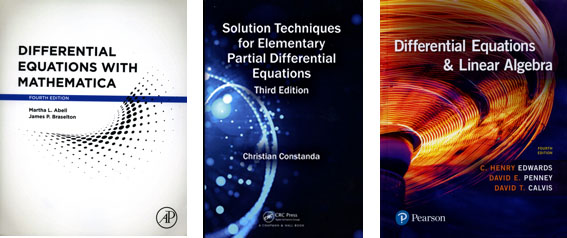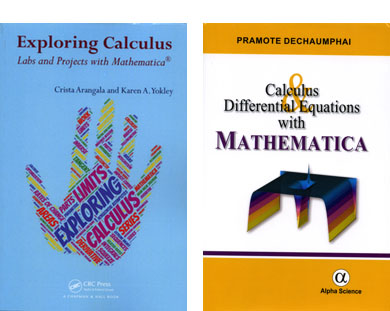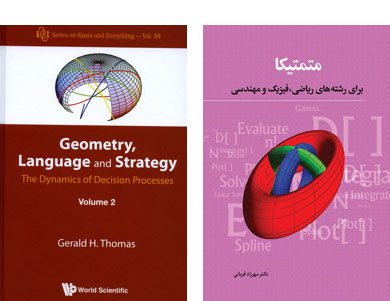Browse by Topic
Related Topics

# New Mathematics Books Utilizing Wolfram Technology

We’re always excited to see how people are using our technology in fields like math and science education, so we keep an eye out for new books that give educators ideas about exploring computational thinking in their classrooms. Here are a few titles we’ve come across recently. These books range from highly theoretical mathematical explorations in the Wolfram Language to Mathematica labs for studying calculus.## New Editions with Additional Mathematica Content

The fourth edition of Differential Equations with Mathematica is a supplementing reference that uses the fundamental concepts of Mathematica to solve (analytically, numerically and/or graphically) differential equations of interest to students, instructors and scientists. Authors Martha L. Abell and James P. Braselton include instruction on basic methods and algorithms. They cover the Mathematica functions relevant to differential equations and dependant concepts from calculus and linear algebra. This book contains many helpful illustrations that make use of Mathematica’s visualization capabilities.

Solution Techniques for Elementary Partial Differential Equations, Third Edition

Christian Constanda teaches students to solve partial differential equations through concise, easily understood explanations and worked examples that allow students to see the techniques in action. The third edition includes new sections on series expansions of more general functions, other problems of general second-order linear equations, vibrating string with other types of boundary conditions and equilibrium temperature in an infinite strip. It also includes new and improved exercises with a brief Mathematica program for nearly all of the worked examples, teaching students how to verify their results with a computer.

Differential Equations & Linear Algebra, Fourth Edition

Authors C. Henry Edwards, David E. Penney and David Calvis provide updated and improved figures, examples, problems and applications. With real-world applications and a blend of algebraic and geometric approaches, Differential Equations & Linear Algebra introduces students to mathematical modeling of real-world phenomena and offers an array of problem sets. Alongside this fourth edition, an expanded applications website is now available that includes programming tools from Mathematica and Wolfram|Alpha.## New Titles That Give Students Hands-on Experience with Mathematica

Exploring Calculus: Labs and Projects with Mathematica
Authors Crista Arangala and Karen A. Yokley created a hands-on lab manual that can be used in class every day to guide the exploration of the theory and applications of differential and integral calculus. Each lab consists of an explanation of material with integrated exercises. The exercise sections integrate problems, technology, Mathematica R visualization and the Computable Document Format (CDF) to help students discover the theory and applications of differential and integral calculus in a meaningful and memorable way.

Calculus and Differential Equations with Mathematica

In this book, Pramote Dechaumphai offers a clear and easy-to-understand presentation of how to use Mathematica to solve calculus and differential equation problems. It contains essential topics that are taught in calculus and differential equation courses, including differentiation, integration, ordinary differential equations and Laplace and Fourier transforms, as well as special functions normally encountered in solving science and engineering problems. Numerical methods are employed when the exact solutions are not available. Additionally, the finite element method in Mathematica is used to analyze partial differential equations for problems with complex geometry. These partial differential equations could be in elliptic, parabolic and hyperbolic forms. Many examples are presented with detailed derivation for their solutions before using Mathematica to confirm the results.## New Titles Exploring Theory with Mathematica

The first volume, Geometry, Language and Strategy, extended the concepts of game theory, replacing static equilibrium with a deterministic dynamic theory. It also opened up many applications that were only briefly touched on. To study the consequences of the deterministic approach and the extent of these applications in contrast to standard Bayesian approaches requires an engineering foundation and discipline, which this volume supplies. It provides a richer list of applications, such as the prisoner’s dilemma, expanding the relevance of volume 1 to more general time-dependent and transient behaviors.

Mathematica for Mathematics, Physics and Engineers

Mehrzad Ghorbani expands on an earlier work, Applied Mathematical Softwares: Mathematica, developed over the course of more than 10 years of teaching mathematics software and Mathematica code in Iranian universities. This new title includes more elegant and basic mathematical problems from a range of specializations including calculus, number theory, numerical analysis, vector and matrix algebra, complex variables, graph theory, engineering mathematics and mathematical physics. Although applicable to undergraduate and graduate studies in math and science, Ghorbani’s book is additionally relevant to those who use Mathematica in computational scientific branches that need symbolic or numerical code.